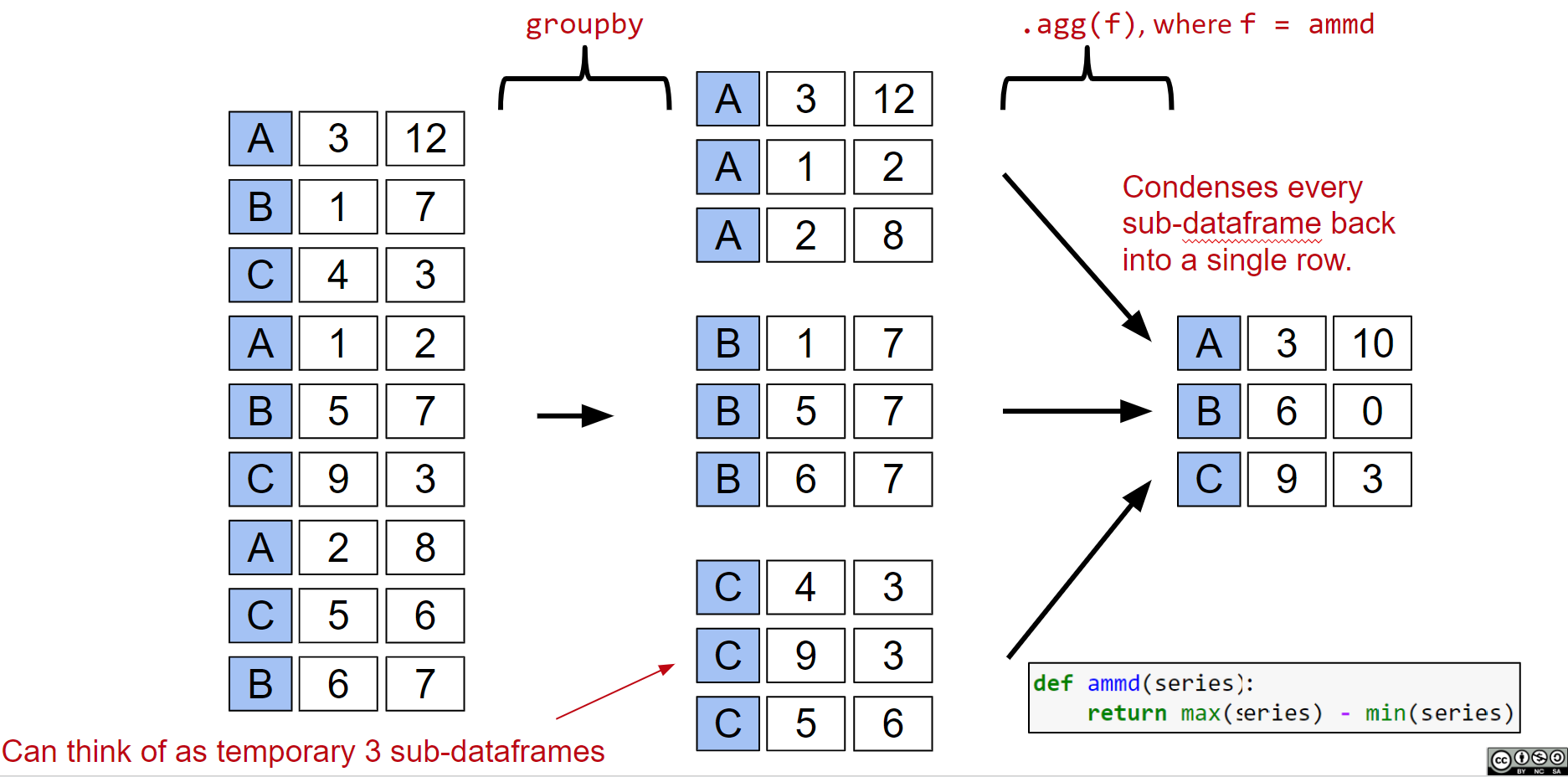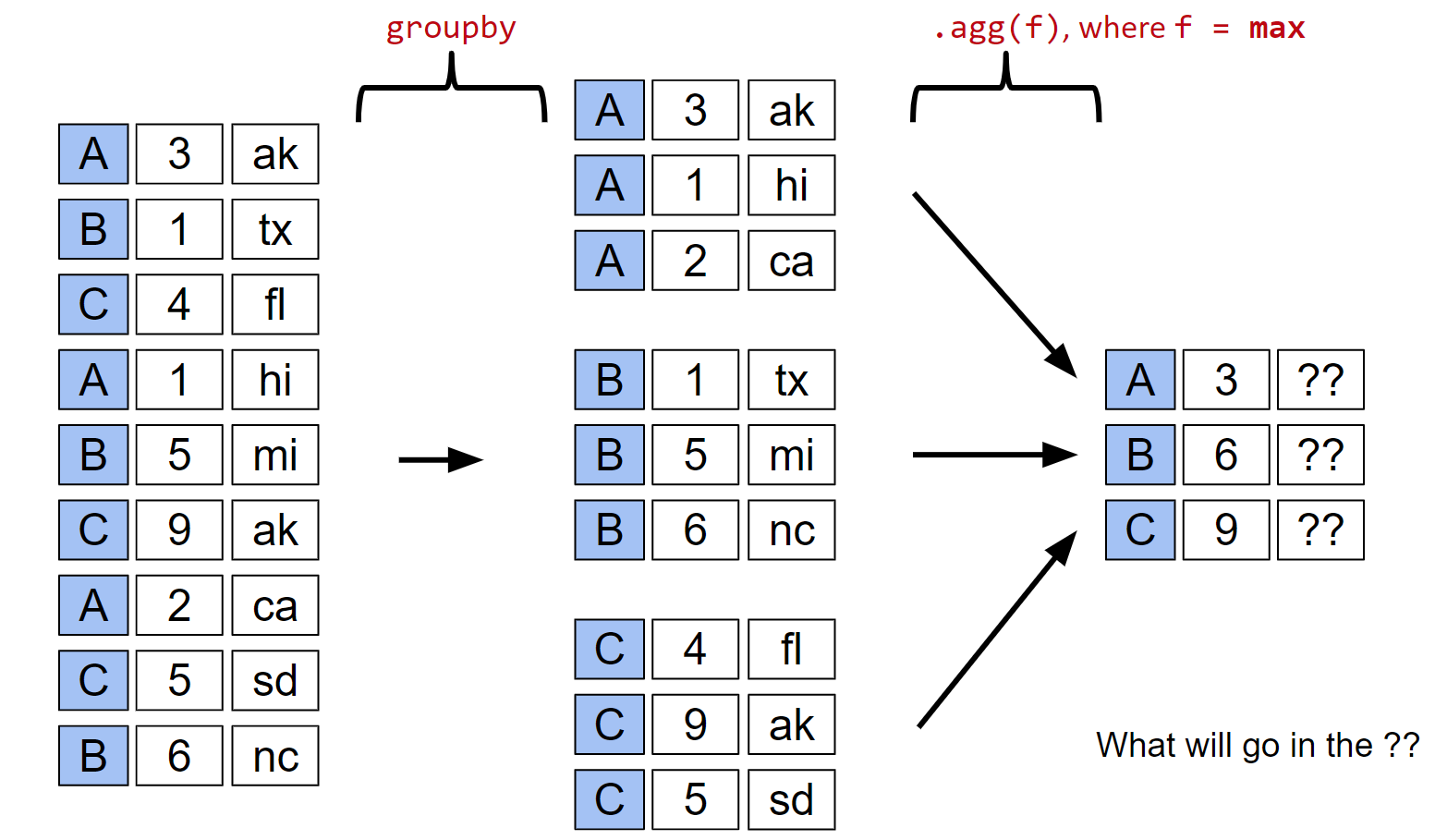Goal 1: Find the most popular baby name in California in 2018

### Approach 2: Use the Series.str methods.¶

In-lecture challenge: Try to write a line of code that creates a list (or Series or array) of all names that end with “ert”.

## Goal 3: Sort names by their length.¶

Suppose we want to sort all baby names in California by their length.

As before, there are ways to do this using only lecture 5 content. For example, the montrosity below was concocted during the Sp19 version of this class.

### Approach 1: Create a temporary column.¶

Create a new series of only the lengths. Then add that series to the dataframe as a column. Then sort by that column. Then drop that column.

We can also use the Python map function if we want to use an arbitrarily defined function. Suppose we want to sort by the number of occurrences of "dr" plus the number of occurences of "ea".

### Approach 2: Generate an index sorted in the desired order.¶

Another approach is to take advantage of the fact that .loc can accept an index. That is:

• df.loc[idx] returns df with its rows in the same order as the given index.
• Only works if the index exactly matches the DataFrame.

The first step was to create a sequence of the lengths of the names.

The next step is to sort the new series we just created.

Next, we pass the index of the sorted series to the loc method of the original dataframe.

Note we can also do this all in one line:

### Goal 4: Name whose popularity has changed the most.¶

First we need to define change in popularity.

For the purposes of lecture, let’s stay simple and use the AMMD (absolute max/min difference): max(count) - min(count).

To make sure we understand this quantity, let's consider the name Jennifer.

The AMMD for Jennifer is 6,061, as seen below:

### Approach 1: Naive For Loop¶

As a first approach, we can try to use a for loop.

Answer below. Note that we only used the first 100 names because otherwise the code takes ages to complete running.

### Approach 2: Use groupby.agg¶

Instead, we can use the very powerful groupby.agg operation, which allows us to simply and efficiently compute what we want.

Note that the result includes both a Year and Count column. The Count column is what we want, namely the AMMD for the name in that row. To check your understanding, try to figure out what the Year column represents.

To understand how groupby works, consider the visual diagram below. The groupby function clusters rows from the original dataframe into groups (which I call subframes). The agg function then condenses each subframe into a single representative row using the provided function f.Groupby puzzle #1: To test your understanding, try to interpret the result of the code below.

For reference, the first 5 values from the plot above are:

#### groupby Puzzle #2¶

We have to be careful when using aggregation functions. For example, the code below might be misinterpreted to say that Woodrow Wilson ran for election in 2016. Why is this happening?

### groupby puzzle #3¶

Inspired by above, try to predict the results of the groupby operation shown. The answer is below the image.The top ?? will be "hi", the second ?? will be "tx", and the third ?? will be "sd".

### groupby puzzle #4¶

Next we'll write code that properly returns the best result by each party. That is, each row should show the Year, Candidate, Popular Vote, Result, and % for the election in which that party saw its best results (rather than mixing them as in the example above).

### groupby puzzle #6: Total Male and Female babies born¶

Suppose we want to find the total number of male and female babies born each year in California.

Try to figure out how we'd do this using groupby.

## Other groupby Features¶

It is possible to group a DataFrame by multiple features. For example, if we group by Year and Sex we get back a DataFrame with the total number of babies of each sex born in each year.

The DataFrame resulting from the aggregation operation is now multi-indexed. That is, it has more than one dimension to its index. We will explore this in next week's exercises.

### groupby.sum(), groupby.mean(), etc.¶

As an alternative to groupby.agg(sum), we can also simply do groupby.sum().

The same applies for many other common operations.

## Pivot Tables¶

### Goal 5: Finding the number of babies born in each year of each sex.¶

Suppose we want to build a table showing the total number of babies born of each sex in each year. One way is to groupby using both columns of interest.

A more natural approach is to use a pivot table (like we saw in data 8).

The basic idea behind pivot tables is shown in the image below.